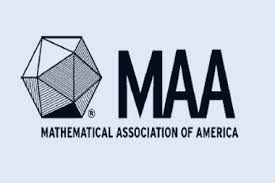# AIME Problem 2

Probability Level 4The nine delagates to the Economic Cooperation Conference include 2 officials from Mexico, 3 officials from Canada, and 4 officials from the United States. During the opening session, three of the delegates fall asleep. Assuming that the three sleepers were determined randomly, the probability that exactly two of the sleepers are from the same country is $\tfrac{m}{n}$, where $m$ and $n$ are relatively prime positive integers. Find $m+n$.

This problem is part of this set.

×